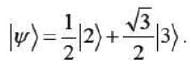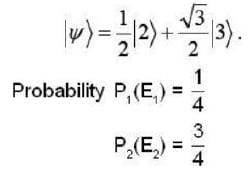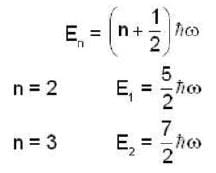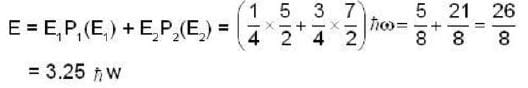A one dimensional harmonic oscillator is in t...
A one dimensional harmonic oscillator is in the superposition of number states, |nn, given byThe average energy of the oscillator in the given state is __________ hw.
A one dimensional harmonic oscillator is in the superposition of numbe...
A one dimensional harmonic oscillator is in the superposition of number states |nn, given byThe energy of the |nn state in one dimensional harmonic oscillatorTotal energy1 Crore+ students have signed up on EduRev. Have you?
1 Crore+ students have signed up on EduRev. Have you?

### Learn this topic in detailGATE Physics Mock Test Series - 8 60 Ques | 180 MinsView courses related to this question

### Quick links for GATE exam850+
Video Lectures2500+
Revision Notes600+
Online Tests34+
Courses
A one dimensional harmonic oscillator is in the superposition of number states, |nn, given byThe average energy of the oscillator in the given state is __________ hw.Correct answer is '3.25'. Can you explain this answer?
Question Description
A one dimensional harmonic oscillator is in the superposition of number states, |nn, given byThe average energy of the oscillator in the given state is __________ hw.Correct answer is '3.25'. Can you explain this answer? for GATE 2023 is part of GATE preparation. The Question and answers have been prepared according to the GATE exam syllabus. Information about A one dimensional harmonic oscillator is in the superposition of number states, |nn, given byThe average energy of the oscillator in the given state is __________ hw.Correct answer is '3.25'. Can you explain this answer? covers all topics & solutions for GATE 2023 Exam. Find important definitions, questions, meanings, examples, exercises and tests below for A one dimensional harmonic oscillator is in the superposition of number states, |nn, given byThe average energy of the oscillator in the given state is __________ hw.Correct answer is '3.25'. Can you explain this answer?.
Solutions for A one dimensional harmonic oscillator is in the superposition of number states, |nn, given byThe average energy of the oscillator in the given state is __________ hw.Correct answer is '3.25'. Can you explain this answer? in English & in Hindi are available as part of our courses for GATE. Download more important topics, notes, lectures and mock test series for GATE Exam by signing up for free.
Here you can find the meaning of A one dimensional harmonic oscillator is in the superposition of number states, |nn, given byThe average energy of the oscillator in the given state is __________ hw.Correct answer is '3.25'. Can you explain this answer? defined & explained in the simplest way possible. Besides giving the explanation of A one dimensional harmonic oscillator is in the superposition of number states, |nn, given byThe average energy of the oscillator in the given state is __________ hw.Correct answer is '3.25'. Can you explain this answer?, a detailed solution for A one dimensional harmonic oscillator is in the superposition of number states, |nn, given byThe average energy of the oscillator in the given state is __________ hw.Correct answer is '3.25'. Can you explain this answer? has been provided alongside types of A one dimensional harmonic oscillator is in the superposition of number states, |nn, given byThe average energy of the oscillator in the given state is __________ hw.Correct answer is '3.25'. Can you explain this answer? theory, EduRev gives you an ample number of questions to practice A one dimensional harmonic oscillator is in the superposition of number states, |nn, given byThe average energy of the oscillator in the given state is __________ hw.Correct answer is '3.25'. Can you explain this answer? tests, examples and also practice GATE tests.(Scan QR code)